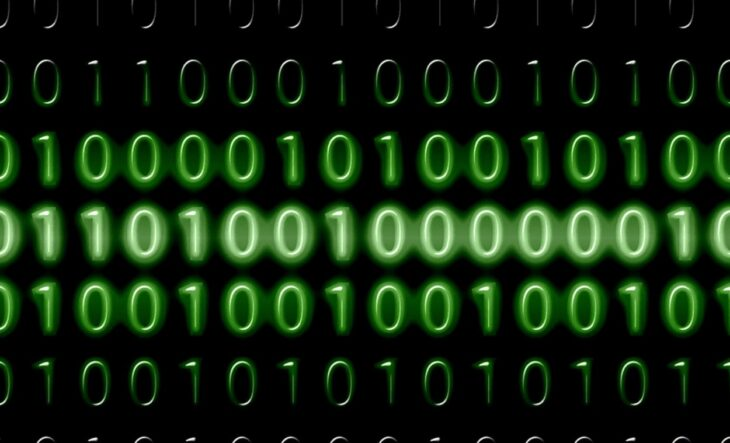# Binary Numbers – Beginner’s Guide 2023

If the above figure makes you nostalgic, I’m certain that you’ve played the Binary sudoku before! Remember the last pages of your school notebooks? Those days were fun, weren’t they?

Binary is the underpinning of current computer technology. The binary number system plays a huge role in the process of  ‘Digital Encoding.’ Thus the Binary Numbers play a huge role in connecting people all around the world through computers.

• Binary Numbers – Beginner’s Guide
• Overview of Base 10 System or Decimal System
• Binary number system
• Decimal number system
• Binary to Decimal Conversions
• Decimal to Binary Conversions
• Examples of Hexadecimal Numbers & its conversions
• Conclusion

## Overview of Base 10 System or Decimal System

The base 10 system is also known as the Decimal system. Base-10 is used in most modern civilizations and was also common for ancient civilizations, most likely because humans have 10 fingers!

A decimal is any number in our base-ten number system. The decimal point is used to separate the ones place from the tenths place in decimals. As we move to the right of the decimal point, each number place is divided by 10. When reading decimals, the decimal point should be read as “and.” Thus, we read the decimal 57.49 as “fifty-seven and forty-nine hundredths.

With the advent of e-technology binary systems emerged as a counting system to communicate with computers. It has a wide range of applications in the real world. Let’s explore how the binary system works.

Let’s get started!

### Binary number system

• The binary number system uses only two digits: 0 and 1.
• Thus, the numbers in this system have a base 2.
• 0 and 1 are called bits.
• 8 bits together make a byte.
• The data in computers is stored in the form of bits and bytes.

Examples: 10112, 10010012,1112, etc.

Application: The binary number system is used in computer technology. All computer languages and programs are based on the 2-digit number system used in digital encoding ( the process of representing data as discrete bits of information).

### Decimal number system

• The decimal number system uses ten digits: 0, 1, 2, 3, 4, 5, 6, 7, 8, and 9.
• Thus, the numbers in this system have a base of 10.
• If we see a number without a base, it means that its base is 10.
• This is the system that we generally use to represent numbers in real life.

Examples: 1810, 22210, 46710, 787710

Applications:  We use decimals everyday when dealing with money, weight, length, etc. Decimal numbers are used in situations where more precision is required than the whole numbers can provide.

### Binary to Decimal Conversions

Multiply each digit of the given number, starting from the rightmost digit, with the exponents of the base 2, where the exponents start from 0 and increase by 1 every time. Then just add the above products.

Let’s try an example.

Example:

Convert 10000102 to the decimal system.

Solution:

Starting from the rightmost digit, we multiply each digit with the corresponding powers of 2. Let’s start.

Adding all the numbers, we get 0+2+0+0+0+0+64 = 66

Pictorially,

Thus,

10000102 = 6610

### Decimal to Binary Conversions

Divide the given number by the base of the required number and note down the quotient and the remainder in the “quotient-remainder” form repetitively until we get the quotient to be less than the base. The new number in the required number system is obtained just by reading all the remainders and the last quotient from bottom to top.

Let’s try an example.

Example:

Convert 99910 to binary system.

Solution:

Reading all the remainders along with the last quotient from bottom to top we get the required conversion.

Thus,

99910 = 11111001112

• The hexadecimal number system uses sixteen digits/alphabets: The digits 0, 1, 2, 3, 4, 5, 6, 7, 8, 9, and the alphabets A, B, C, D, E, and F.
• Thus, the numbers in this system have a base of 16.
• Here, A−F of the hexadecimal system indicates the numbers 10−15 of the decimal number system, respectively.
• This system is used in computers to reduce the large-sized strings of the binary system.

Examples: A7B16, BC16, etc.

Applications: A computer can understand positional number systems where there are only a few symbols called digits, and these symbols represent different values depending on the position they occupy in the number. Computers are usually designed to process hexadecimal numbers.

### Examples of Hexadecimal Numbers & its conversions

Example 1:

Convert 16F80E16 into the binary system.

Solution:

Conversion of 16F80E16 to decimal: Multiply each digit with corresponding powers of 16, starting from the rightmost digit.

Adding all the numbers, we get  14+0+2048+61440+393216+1048576 = 1505294

So 16F80E16 = 150529410

Conversion of 150529410 to binary:

Reading all the remainders along with the last quotient from bottom to top, we get the required conversion.

Thus,

150529410 = 1011011111000000011102

Hence, 16F80E16 = 1011011111000000011102

Example 2:

Convert 1000112  into the hexadecimal system.

Solution:

To convert a binary into a hexadecimal number first, we have to convert the binary into decimal and then from decimal to hexadecimal.

Conversion of 1000112 to decimal: Multiply each digit with corresponding powers of 2, starting from the rightmost digit.

Adding all the numbers, we get  1+2+32 = 35

So, 1000112 = 3510

Reading all the remainders along with the last quotient from bottom to top, we get the required conversion.

Thus,

3510 = 2316

Hence, 1000112 = 2316